Name:    Math Challengers Quiz 5.1 Chords

Multiple Choice
Identify the choice that best completes the statement or answers the question.

1.

What is the length of AO?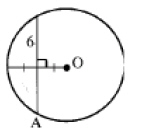a.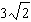c. 3.4 b. 3.5 d.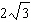2.

What is the length of AB if CD=2 and ED =8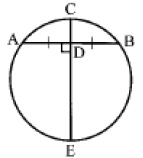a.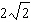c. 8 b.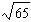d. 10

3.

What is the length of AB?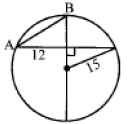a.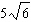c. 17 b.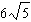d. 19

4.

Find the length of CD:
Given that AB=10, CF=21, and AE=8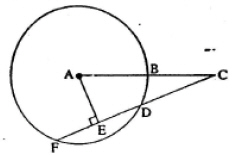a.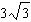c. 9 b.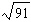d. 12

5.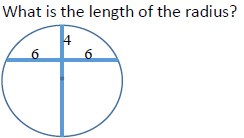a. 6.5 c. 7.5 b. 7 d.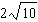6.

If the radius of the smaller circle is 2 and the radius of the larger circle is 5, what is the distance between the two centres of each circle? Note: the intersection of the two tangents is perpendicular: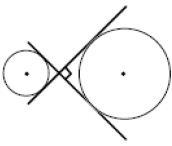a. 7 c.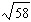b.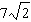d.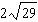7.

Find the area of the shaded region: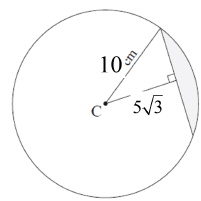a.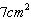c.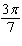b.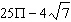d.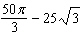8.

Given the semi circle and that AB is a diameter, what is the length of PQ?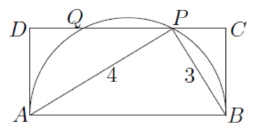a. 2.3 c. 2.8 b. 1.4 d. 1.5

9.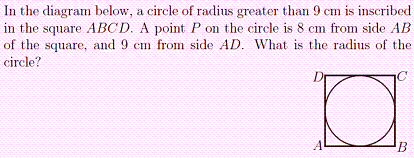a. 12 c. 29 b. 28 d. 30

10.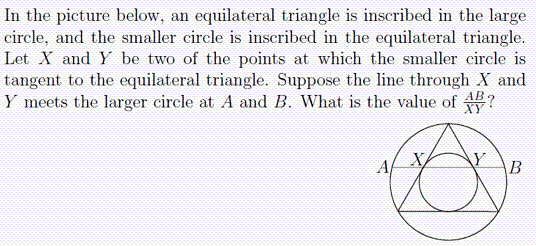a.c. 2 b.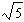d.